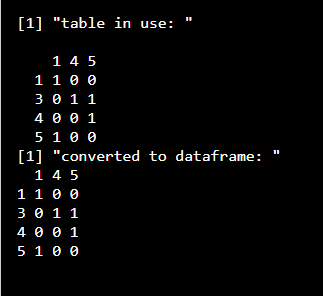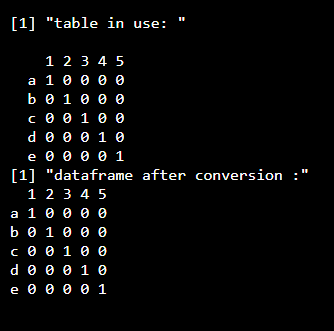GeeksforGeeks App
Open AppBrowser
Continue

# How to convert table to dataframe in R?

In this article, we will discuss how to convert a given table to a dataframe in the R programming language.

### Functions Used

• as.data.frame.matrix() will be taking the table as its parameter and will return the dataframe back to the user.

Syntax: as.data.frame.matrix(x)

Parameter:

• x: name of the table which is to be converted into dataframe

Returns: It will return the dataframe which is converted from the given data.

• table() function is used for the cross-classifying factors to build a contingency table of the counts at each combination of factor levels of the provided data in R language.

Syntax: table(…,exclude = if (useNA == “no”) c(NA, NaN),useNA = c(“no”, “ifany”, “always”),dnn = list.names(…), deparse.level = 1)

Returns:

This function will be returning the frequency table of the provided data back to the user.

Thus, we will first create a contingency table using table() function from a dataframe and then convert this table to a dataframe.

Given below are some implementations for this.

Example 1:

## R

 `data_gfg=``data.frame``(x=``c``(1,3,5,3,4),``                    ``y=``c``(1,5,1,4,5))` `table_gfg=``table``(data_gfg\$x,data_gfg\$y)``print``(``"table in use: "``)``table_gfg` `new_data_gfg=``as.data.frame.matrix``(table_gfg)``print``(``"converted to dataframe: "``)``new_data_gfg`

Output:Example 2:

## R

 `data_gfg=``data.frame``(x=``c``(``"a"``,``"b"``,``"c"``,``"d"``,``"e"``),``                    ``y=``c``(``"1"``,``"2"``,``"3"``,``"4"``,``"5"``))` `table_gfg=``table``(data_gfg\$x,data_gfg\$y)``print``(``"table in use: "``)``table_gfg` `new_data_gfg=``as.data.frame.matrix``(table_gfg)``print``(``"dataframe after conversion :"``)``new_data_gfg`

Output:My Personal Notes arrow_drop_up